# Online Electronics and Communication Engineering Test - Digital Electronics Test 2Loading Test...

Instruction:

• This is a FREE online test. DO NOT pay money to anyone to attend this test.
• Total number of questions : 20.
• Time alloted : 30 minutes.
• Each question carry 1 mark, no negative marks.
• DO NOT refresh the page.
• All the best :-).

1.

An 8 bit data is to be entered into a parallel in register. The number of clock pulses required is

A.
 8B.
 4C.
 2D.
 1Explanation:

In a parallel in register only one pulse is needed to enter data.

2.

When microprocessor processes both positive and negative numbers, the representation used is

A.
 l's complementB.
 2's complementC.
 signed binaryD.
 any of the aboveExplanation:

2's complement representation requires simple electronic circuitry.

3.

For the logic circuit of the given figure the simplified Boolean expression is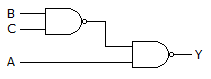A.
 A + BCB.
 A + BCC.
 A + B CD.
 A + BCExplanation: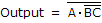= A + BC.

4.

718 = __________ .

A.
 1110002B.
 1110012C.
 1000012D.
 1100012Explanation:

71 in octal = 7 x 8 + 1= 57 in decimal = 111001 in binary.

5.

In the TTL circuit in the figure, S2 to S0 are select lines and X7 to X0 are input lines. S0 and X0 are LSBs. The output Y is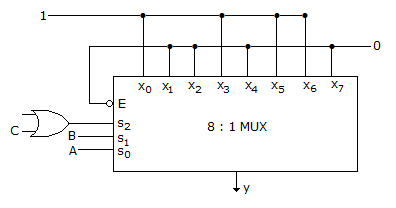A.
 IndeterminateB.
 A ⊕ BC.
 A ⊕ BD.
 C . A ⊕ B + C . (A ⊕ B)Explanation:

The MUX is made up of TTL circuit. For TTL circuit open terminal is taken high, since S2 select line is connected to OR gate whose one terminal connected to C and the other is open (high) so OR gate output is S2 = 1 + C = 1.

```S2 = 1 S1(B) S0(A)  Y
1       0     0     0
1       0     1     1
1       1     0     1
1       1     1     0```

Y = S0⊕S1 => A⊕B.

6.

How many JK flip-flops are needed to make a 4-bit shift register?

A.
 2B.
 4C.
 6D.
 87.

A compiler is a software programme which

A.
 change high level Programming language into low level machine languageB.
 change the program into binary formC.
 assembles the programD.
 executes the program8.

A.
 two, threeB.
 three, fourC.
 four, sixD.
 two, four9.

A 555 timer can be used as

A.
 an astable multivibratorB.
 monostable multivibratorC.
 frequency Divider onlyD.
 any of the above10.

The Boolean function A + BC is reduced form of

A.
 AB + BCB.
 AB + ABCC.
 (A + B) (A + C)D.
 (A + C)B11.

In a Karnaugh map for an expression having 'don't care terms' the don't cares can be treated as

A.
 0B.
 1C.
 1 or 0D.
 none of the above12.

Which of the following is coincidence logic circuit?

A.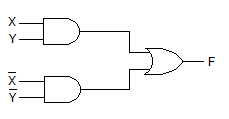B.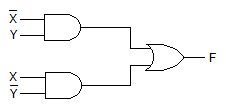C.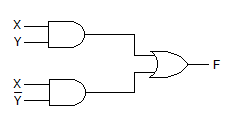D.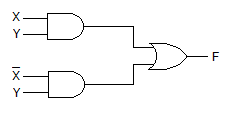13.

The circuit in the given figure is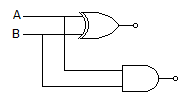A.
 half adderB.
 full adderC.
 half subtractorD.
 full subtractor14.

A.
 two 4-bit binary numbersB.
 four 6-bit binary numbersC.
 four 8-bit binary numbersD.
 any number of 4-bit numbers15.

Mark the false statement:

A.
 since wave-shape of a digital signal is unimportant, linear amplifiers are not requiredB.
 the precise amplitude of a digital signal is also unimportant provided two voltage levels (0 and 1) are quite differentC.
 the leading and trailing edges of a digital signal need not be sharpD.
 actual digital waves have rise and fall times of the order of a few nano seconds16.

The address to which a software or hardware restart branches is known as

A.
 Vector locationB.
 SIDC.
 SODD.
 TRAP17.

Consider the following digital circuits: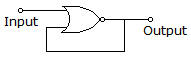1. Multipliers
3. D-latch
4. Circuits as shown
Which of these come under the class of combinational circuit?

A.
 1 and 2B.
 3 and 4C.
 1, 2 and 3D.
 1, 2, 3 and 418.

A 16 x 8 ROM stores these words in its first four locations, as given below. Which of this represents 3 CH in hexadecimal?

A.
 R0 = 1110 0010B.
 R1 = 0101 0111C.
 R2 = 0011 1100D.
 R3 = 1011 111119.

What is the maximum output voltage from the 741 op-amp?

A.
 ZeroB.
 Approximately 1 VC.
 Approximately 3 VD.
 Approximately 5 V20.

Assuming accumulator contain A 64 and the carry is set (1). What will register A and (CY) contain after ACI 0 A4 H?

A.
 4 AH, 0B.
 4 AH, 1C.
 4 BH, 0D.
 4 BH, 1